Sample Size Calculator: Confidence Interval – Bristol

Hypothesis: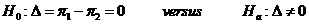Data Input: (Help) (Example)

 Input Results α P1 n P2 N L0

Note:

 Variables Descriptions α Significance level P1 Observed proportion of success in arm 1 P2 Observed proportion of success in arm 2 L0 A specific positive value, bound of expected length of 100(1- α)% confidence interval n Sample size per arm N Total sample size

Help Aids

Application:  The determination of the sample size to control the power and to control the length of the confidence interval, following hypotheses are usually used:where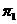and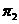denote the success probabilities of interest.

Procedure:

1. Enter

a)   value of α, the probability of type I error

b)   value of P1, the observed proportion of success in arm 1

c)   value of P2, the observed proportion of success in arm 2

d)   value of L0, the bound of expected length of 100(1- α)% confidence interval.

1. Click the button “Calculate” to obtain

a)    value of n, the sample size per arm

b)    value of N, the total sample size

Formula:

Let nL donotes the sample size required to have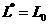, a specified positive value.

where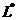is the expected length,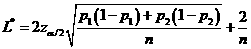, of (1- α) confidence interval of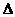.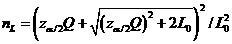where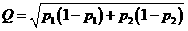,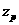is the upper 100(1-p) percentile of the standard normal distribution.

Notations:

α:               The probability of type I error (significance level) is the probability of rejecting the true null hypothesis.

β:               The probability of type II error (1 – power of the test) is the probability of failing to reject the false null hypothesis.

Examples

Example 1:

A clinical trial was planned to compare two active treatments for a certain type of cancer, with respect to their response rates P1 and P2. First consider the hypothesis testing formulation, where it is planned to test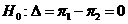against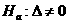at 5% significance level (α=0.05). The sample size is chosen such that the power is 0.80 when the smaller response rate is 0.20 (P1=0.20) and the larger response rate is 0.30 (P2=0.30). For these values of P1 and P2, the approximation to the expected length of the 95% confidence interval is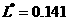. The sample size required to guarantee that the approximation to the expected length of the 95% confidence interval is at most L0=0.2 is 162 patients per treatment (n=162). Total sample size required is 324 (N=324).

Reference: Bristol, David R. "Sample Sizes For Constructing Confidence Intervals and Testing Hypothesis." Statistics in Medicine, 8 (1988):803-811. Print.